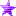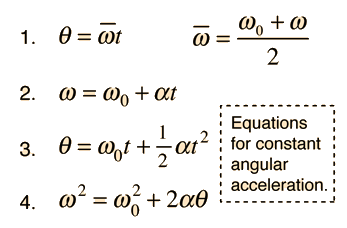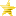# Outerra forum

• December 10, 2023, 07:32:17 pm• Welcome, Guest

Pages: 

###AuthorTopic: Auto-targeting turrets  (Read 5911 times)

#### zzz

• Sr. Member
•• Posts: 266
• newbie##### Auto-targeting turrets
« on: August 17, 2015, 07:24:57 pm »

http://www.moddb.com/games/eveworld/videos/turret-auto-targeting

Sharing this code. Hoping to get some help developing it.

Code: [Select]
`//Multiplies matrix m1 and m2 togetherfunction multiplyMatrices(m1, m2) {    var result = [];    for (var i = 0; i < m1.length; i++) {        result[i] = [];        for (var j = 0; j < m2.length; j++) {            var sum = 0;            for (var k = 0; k < m1.length; k++) {                sum += m1[i][k] * m2[k][j];            }            result[i][j] = sum;        }    }    return result;}//Rotates the axis of originalCoordinates through the xyz angles inputted to produce coordinates aligned with those anglesfunction rotateECEF(xangle, yangle, zangle, origCoords) { var A1 = [[Math.cos(yangle)*Math.cos(zangle), -Math.cos(yangle)*Math.sin(zangle), Math.sin(yangle), 0], [Math.cos(xangle)*Math.sin(zangle) + Math.sin(xangle)*Math.sin(yangle)*Math.cos(zangle), Math.cos(xangle)*Math.cos(zangle) - Math.sin(xangle)*Math.sin(yangle)*Math.sin(zangle), -Math.sin(xangle)*Math.cos(yangle), 0], [Math.sin(xangle)*Math.sin(zangle) - Math.cos(xangle)*Math.sin(yangle)*Math.cos(zangle), Math.sin(xangle)*Math.cos(zangle) + Math.cos(xangle)*Math.sin(yangle)*Math.sin(zangle), Math.cos(xangle)*Math.cos(yangle), 0], [0, 0, 0, 1]]; return multiplyMatrices(A1, origCoords);}//Calculate the angles between the origin and a set of coordinates, allowing for the target's speed and the time required for the bullet to reach its destination. Returns the yaw and pitch for the turret.function aimAngle(targetPos, targetVelo, bulletSpeed) {    var rCrossVYaw = targetPos * targetVelo - targetPos * targetVelo;    var magRYaw = Math.sqrt(targetPos*targetPos + targetPos*targetPos);    var rCrossVPitch = magRYaw * targetVelo - targetPos *  Math.sqrt(targetVelo*targetVelo + targetVelo*targetVelo);    var magRPitch = Math.sqrt(magRYaw*magRYaw + targetPos*targetPos);    var angleAdjustYaw = Math.asin(rCrossVYaw / (bulletSpeed * magRYaw));    var angleAdjustPitch = Math.asin(rCrossVPitch / (bulletSpeed * magRPitch));    return [-(angleAdjustYaw + Math.atan2(targetPos, targetPos)), -(angleAdjustPitch + Math.atan2(targetPos, magRYaw))];}// Calculate the angular distance the turret will travel when it slows from its current speed to zero. Used to stop it overshooting its target angle.function brakeDist(turCurrentSpeed, MaxTurretSpeed, MaxTurretAccel) {  if (turCurrentSpeed > 0) { return (((turCurrentSpeed * MaxTurretSpeed)*((turCurrentSpeed * MaxTurretSpeed)/MaxTurretAccel)) - (0.5 * MaxTurretAccel * (Math.pow(((turCurrentSpeed * MaxTurretSpeed)/MaxTurretAccel), 2)))); } else { return -(((turCurrentSpeed * MaxTurretSpeed)*((turCurrentSpeed * MaxTurretSpeed)/MaxTurretAccel)) - (0.5 * MaxTurretAccel * (Math.pow(((turCurrentSpeed * MaxTurretSpeed)/MaxTurretAccel), 2)))); } }`
Code: [Select]
`// Turret 1 autotarget//First rotate your target coordinates to align with the turret's axis. turZA01 is the roll angle of the turret from Pi to -Pi relative the model, i.e. if your turret is mounted to the right side of your model it is offset -0.25Pi. XA01 is the yaw angle, YA01 is the pitch angle.this.rotTarget01 = rotateECEF(this.heading_pitch_roll().z-turZA01, this.heading_pitch_roll().y-turYA01, -this.heading_pitch_roll().x+turXA01, [[this.targCoordsY], [this.targCoordsX], [this.targCoordsZ], ]);//Get the braking distance for the yaw of the turretthis.yawDist01 = brakeDist(this.turx01.speed, radians(MaxTurretXSpeed01), radians(MaxTurretXAccel01)); //Get the braking distance for the pitch of the turret this.pitchDist01 = brakeDist(this.tury01.speed, radians(MaxTurretYSpeed01), radians(MaxTurretYAccel01));// Make sure the turret's yaw never exceeds range -Pi to Pi  if (this.turx01.value > Math.PI) { this.turx01.value = -Math.PI;} else if (this.turx01.value < -Math.PI) { this.turx01.value = Math.PI;}//Get the yaw and pitch angles the turret needs to match. turY01 is the turret's longitudinal distance from the model's origin, i.e. -20 means the turret is mounted 20m behind the origin. X01 is left/right, Z01 is up/down.this.aim01 = aimAngle([this.rotTarget01 - turY01, this.rotTarget01 - turX01, this.rotTarget01 - turZ01], [0,0,0], MaxTurretProjSpeed01);// Change the turret angle until it matches the desired one. The nested If Statement accounts for the transition from a positive axis to a negative one when it's quicker to rotate from -0.9Pi to 0.9Pi via the 0.2Pi distance rather than the 1.8Pi.if (-this.aim01-this.yawDist01+0.005 < this.turx01.value) { if (Math.abs(this.turx01.value-(-this.aim01-this.yawDist01+0.005)) > Math.PI) { this.turx01.set(1) }else{ this.turx01.set(-1)}} else if (-this.aim01-this.yawDist01-0.005 > this.turx01.value) { if (Math.abs(this.turx01.value-(-this.aim01-this.yawDist01-0.005)) > Math.PI) { this.turx01.set(-1) } else { this.turx01.set(1) }} else { this.turx01.set(0)}// My turrets never pitch through 360 degrees so you'd need to first limit the range and then account for the axis sign change like with the Yaw values above if yours do.if (this.aim01-this.pitchDist01+0.005 < this.tury01.value) { this.tury01.set(-1)} else if (this.aim01-this.pitchDist01-0.005 > this.tury01.value) { this.tury01.set(1)} else { this.tury01.set(0)}  if(this.turx01.changed(dt)){    this.geom.rotate_joint_orig(this.Tur01, this.turx01.value, {z:-1});  }  if(this.tury01.changed(dt)){    this.geom.rotate_joint_orig(this.Gun01, this.tury01.value, {x:1});  }`
A target at (200, 0, 50) is a target 200m right of the turret, 50m above. Try with a turret that is upright and centered so you can set all offsets to 0 for first timers.

Some current issues:

1. Fairly certain there are some double negatives in there.

2. You'll notice some x/y/z angles are assigned to variables out of order or negatively. The angles in the tutorial code I looked at differed from the angle set used in Outerra.

3. The angular displacement distance is based on these equationsCode: [Select]
`this.yawSpeed01 = (this.turx01.speed * radians(MaxTurretXSpeed01));this.yawTime01 = this.yawSpeed01/radians(MaxTurretXAccel01);this.yawDist01 = (this.yawSpeed01*this.yawTime01) - (0.5*radians(MaxTurretXAccel01)*(Math.pow(this.yawTime01, 2)));`
However for low acceleration values the distance is incorrect. I also have to add small values like the 0.005s above to stop it from vibrating.

4. Very, very occasionally matrix values get treated as strings, and only when adding something. So 2+15 becomes 215 instead of 17. Especially with nested values such as "var test = [, , ]". I come from a Matlab background so to ensure a vertical matrix for correct multiplication I write it like that.

5. I would like to change all the label numbers (01 in the quoted code) to matrix indexes so that for a vehicle with lots of turrets I can do a For Loop instead of copying and pasting N times. Keep getting errors in Outerra when declaring them.

Finally the biggest thing I'd like help with is modifying it to let objects navigate to ECEF coordinates. Looking at the differences in axis between ECEF and turrets I found that:

Y axis = ECEF's X axis
X axis = ECEF's negative Y axis
Z = Z

For angles Longitude is the same as the turret's negative X axis, Latitude is the turret's negative Y axis offset 90 degrees (kinda fuzzy). For the time being I've been conducting all my test runs at the North pole so angles come into it less.

So if we treat the vehicle as a turret and Earth as the vehicle the turret is mounted on, the code should be:

Code: [Select]
`function ecef2lla(pos){ lat = Math.atan2(pos.z, Math.sqrt(pos.x*pos.x + pos.y*pos.y)); lon = Math.atan2(pos.y, pos.x); return [lat, lon];}`
Code: [Select]
`this.lla = ecef2lla(this.geom.get_pos());//Rotate the axisthis.rotDestination = rotateECEF(0, 0, -this.lla, [[this.destX], [-this.destY], [this.destZ], ]);// Get the anglesthis.newHead = aimAngle([this.rotDestination - this.geom.get_pos().x, parseInt(this.rotDestination) + this.geom.get_pos().y, this.rotDestination - this.geom.get_pos().z], [0,0,0], 1);// Yaw axis for models is 0 to 2 Pi in this case, and I need the negative of the returned values.if (this.newHead > 0) { this.newHead = -(2*Math.PI) + this.newHead;}if (-this.newHead-this.yDist-0.005 < this.heading_pitch_roll().x) { this.extra_force({y:100,z:0},{z:0,x:angForce}); this.extra_force({y:-100,z:0},{z:0,x:angForce});} else if (-this.newHead-this.yDist-0.005 > this.heading_pitch_roll().x) { this.extra_force({y:100,z:0},{z:0,x:angForce}); this.extra_force({y:-100,z:0},{z:0,x:angForce});}if (-this.newHead+this.pDist+0.005 < this.heading_pitch_roll().y) { this.extra_force({y:100,z:0},{y:0,z:angForce}); this.extra_force({y:-100,z:0},{y:0,z:angForce});} else if (-this.newHead+this.pDist+0.005 > this.heading_pitch_roll().y) { this.extra_force({y:100,z:0},{y:0,z:angForce}); this.extra_force({y:-100,z:0},{y:0,z:angForce});}// Auto-level code for rollsif (this.heading_pitch_roll().z > this.rDist+0.01) { this.extra_force({z:100,y:0},{z:0,x:angForce}); this.extra_force({z:-100,y:0},{z:0,x:angForce});}if (this.heading_pitch_roll().z < this.rDist+0.01) { this.extra_force({z:100,y:0},{z:0,x:angForce}); this.extra_force({z:-100,y:0},{z:0,x:angForce});}`
The pitch angle is correct but the yaw is not.

Uriah's code (http://forum.outerra.com/index.php?topic=3368.0) calculates the Yaw angle correctly. I tried using his values and my pitch value only, but of course my pitch value is calculated to intersect with my inaccurate XY coordinates.Logged

#### Uriah

• Global Moderator
• Hero Member
•• Posts: 569
•• We do these things not because they are easy. -JFK##### Re: Auto-targeting turrets
« Reply #1 on: August 18, 2015, 12:00:24 am »

Here is the function for elevation angle (pitch):

Code: [Select]
`// calculation elevation (angle) in radians between two sets of ECEF coordinatesfunction elevation_angle (x1, y1, z1, x2, y2, z2) {    var dx = x2 - x1; var dy = y2 - y1; var dz = z2 - z1;    return elevation = Math.acos( (x1*dx + y1*dy + z1*dz) / Math.sqrt((Math.pow(x1,2)+Math.pow(y1,2)+Math.pow(z1,2))*(Math.pow(dx,2)+Math.pow(dy,2)+Math.pow(dz,2))) );}`
Call in update:

Code: [Select]
`  //get world ECEF position offset of bone joint position  pos_ecef = this.geom.get_pos_offset(bone_joint_pos);  //pos_ecef = this.get_pos();  pos_ecef_x = pos_ecef.x;  pos_ecef_y = pos_ecef.y;  pos_ecef_z = pos_ecef.z;  //calculate elevation angle to target position from seeker position ECEF  tgt_ecef_elevation_rad = elevation_angle(    pos_ecef_x,    pos_ecef_y,    pos_ecef_z,    tgt_pos_ecef_x,    tgt_pos_ecef_y,    tgt_pos_ecef_z  );  //calculate elevation angle relative to horizon in degrees  tgt_ecef_elevation_horizon_deg = 90-rad2deg(tgt_ecef_elevation_rad);`
I am working on a function to accurately calculate azimuth between any two ECEF coordinates by transforming between ECEF and the local tangent plane ENU (East, North, Up).

Best regards,
Uriah

http://gis.stackexchange.com/questions/58923/calculate-view-angle

http://www.navipedia.net/index.php/Transformations_between_ECEF_and_ENU_coordinates

http://www.movable-type.co.uk/scripts/latlong.html

http://gis.stackexchange.com/questions/82998/trasformation-from-ecef-to-enu

http://www.apsalin.com/convert-geodetic-to-cartesian.aspx
« Last Edit: August 18, 2015, 12:02:30 am by Uriah »Logged

#### zzz

• Sr. Member
•• Posts: 266
• newbie##### Re: Auto-targeting turrets
« Reply #2 on: August 27, 2015, 07:03:22 am »

I've made some progress on waypointing.

Quote
function ecef2lla(pos){
lat = Math.atan2(pos.z, Math.sqrt(pos.x*pos.x + pos.y*pos.y));
lon = Math.atan2(pos.y, pos.x);

return [lat, lon];
}

function ecef2lla2(pos){
lat = Math.atan2(pos, Math.sqrt(pos*pos + pos*pos));
lon = Math.atan2(pos, pos);

return [lat, lon];
}

//converts ECEF to model's local ENU axis
function ecef2enu(pos, llat, llong) {
t = (Math.cos(llong)*pos) + (Math.sin(llong)*pos);
return [(-Math.sin(llat) * t) + (Math.cos(llat) * pos), (-Math.sin(llong) * pos) + (Math.cos(llong) * pos), (Math.cos(llat) * t) + (Math.sin(llat) * pos)];
}

//get the angle between two points in lat and long
function bear(latA, lonA, latB, lonB) {
X = Math.cos(latB) * Math.sin(lonB-lonA);
Y = (Math.cos(latA) * Math.sin(latB)) - (Math.sin(latA) * Math.cos(latB) * Math.cos(lonB-lonA));
return Math.atan2(X,Y)
}

Quote
this.targCoordsX = 200;
this.targCoordsY = 10;
this.targCoordsZ = 6378500;

this.lla = ecef2lla(this.geom.get_pos());
this.llaTarg = ecef2lla2([this.targCoordsX, this.targCoordsY, this.targCoordsZ])

this.log_inf(this.targCoordsX-this.geom.get_pos().x)
this.log_inf(this.targCoordsY-this.geom.get_pos().y)
this.log_inf(this.targCoordsZ-this.geom.get_pos().z)

this.rotAng = ecef2enu([this.targCoordsX-this.geom.get_pos().x, this.targCoordsY-this.geom.get_pos().y, this.targCoordsZ-this.geom.get_pos().z], this.lla, this.lla)

this.bearing = aimAngle([parseInt(this.rotAng), parseInt(this.rotAng), -parseInt(this.rotAng)], [0,0,0], 1);

if (this.bearing < 0) {
this.bearing = 2*Math.PI+this.bearing;
}

It mostly works, however it results in the ship reaching the X coordinate a second or two before the Y coordinate resulting in a hard turn at the end. Any equations errors immediately stand out?

This code can be used for formation flying where you have a waypoint perpetually ahead of the vehicle, and trailing vehicles using offset waypoints to create parallel paths.Logged
Pages: